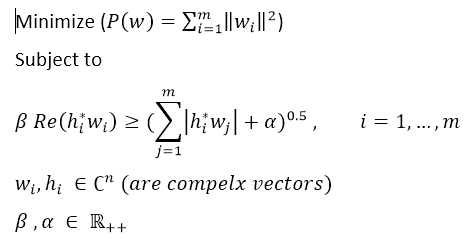# Formulate the following problem please

please help me to write the cvx code for my problemThe non-convexity of the constraint is evident in one dimension. Therefore, it can not be modeled in CVX.

If you think I’m wrong, provide sufficient conditions to prove that.

is this problem a SOCP problem ?
this is a problem from this paper : “A Tractable Method for Robust Downlink Beamforming in Wireless Communication” by : Almir Mutapcic , S-J. kim, and Stephen Boyd.

The paper is at http://web.stanford.edu/~boyd/papers/pdf/rob_downlink_bf.pdf .Lookiing at equation (1), it is evident that there is a typo, specifically, a missing square on the abs(h’w) term in equation (5). With that missing square, it is indeed a SOCP. Without it, as you presented the problem, it is non-convex.

The key step you need is to model a term such as `sqrt(x^2+sigma^2)` as `norm([x sigma])`. With that hint, I’ll let you work it out for this problem. Please read the CVX Users’ Guide first, as this forum is no substitute for doing so. If you use CVX 3.0beta, you can use abs(x) inside the norm.